KNOWLEDGEBASE - ARTICLE #809

# Graph tip - How can I plot an odds ratio plot, also known as a Forest plot, or a meta-analysis plot?This example shows how to make an odds ratio plot (also known as a Forest plot or a meta-analysis plot) which graphs the odds ratios (with 95% confidence intervals) from several studies. It also shows how to place a custom grid line on a graph. This example will assume that the odds ratios (and their 95% confidence intervals) were already calculated and are available for use.

How to do it:

GraphPad Prism makes it easy to create a graph with this type of appearance. Start by creating Grouped data table to enter the data. For this example, we're going to enter values (odds ratios) along with the upper and lower 95% confidence limits associated with those values. To do this, we'll choose the option "Enter and plot error values already calculated elsewhere" for "Y" and select "Mean (or median), Upper/Lower limits" from the dropdown menu.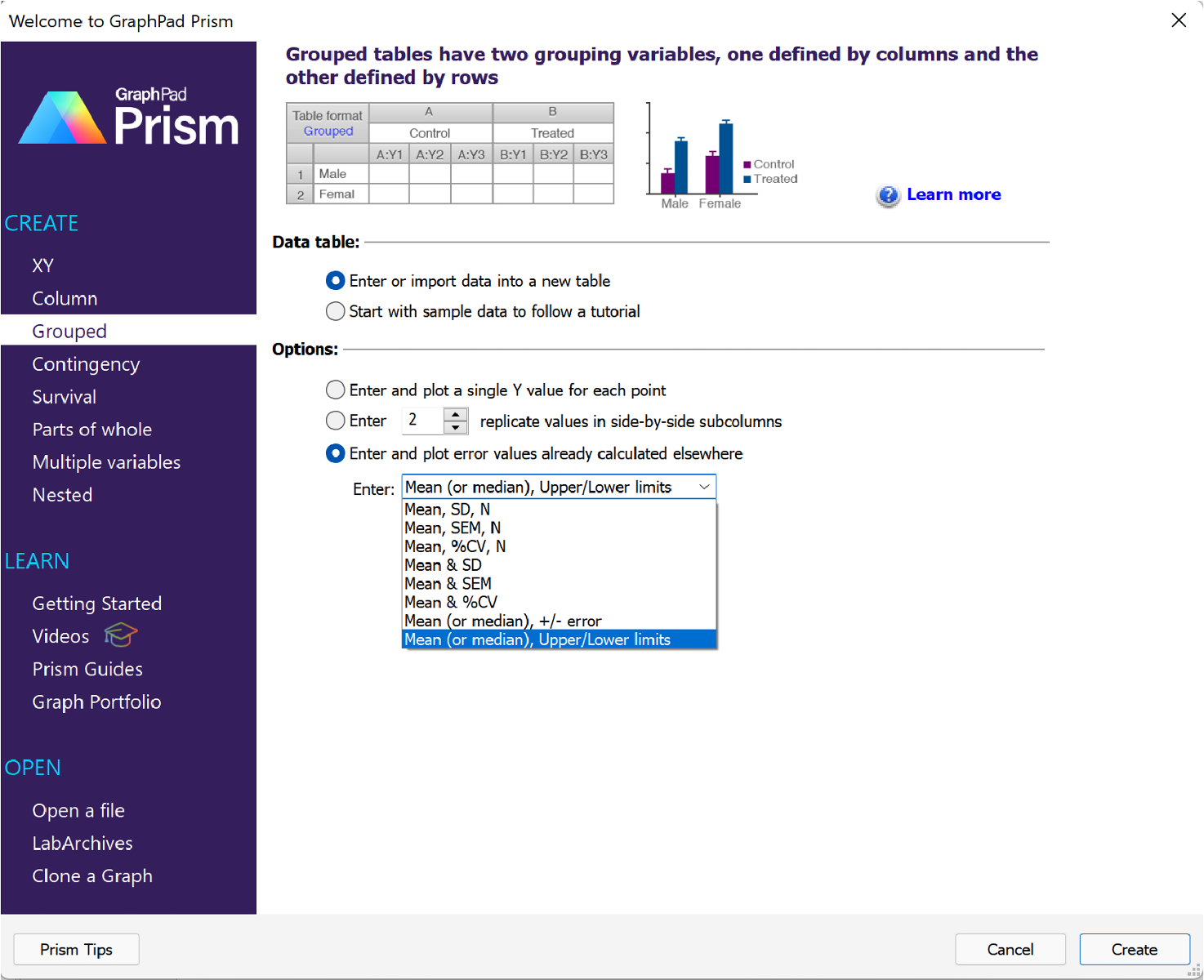After clicking "Create", the empty Grouped data table will be shown and the data can be entered (as shown below). For this example, we're going to enter all of our data into the first column as follows:

• Each row will contain the values (odds ratio, upper confidence limit, and lower confidence limit) from a different study
• The odds ratio will be entered into the first subcolumn
• The upper confidence limit will be entered into the second subcolumn
• The lower confidence limit will be entered into the third subcolumn
• The study names can be entered into the appropriate row titles

Once entered, the data table should look something like this: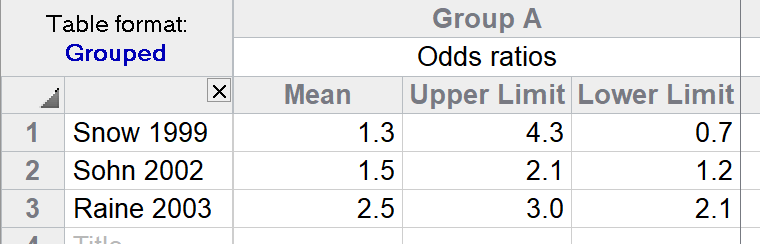Once the data for this first graph have been entered, click on the corresponding graph sheet in the Navigator. Since this is the first time you've viewed the graph, the Change Graph Type dialog will appear so that you can specify the type of graph you'd like to create. For this example, we'll choose the horizontal version of the "Interleaved symbols (at mean or median)" graph type in the Grouped graph family. Make sure that "Mean with error bars" is selected in the "Plot" dropdown menu, and click "OK".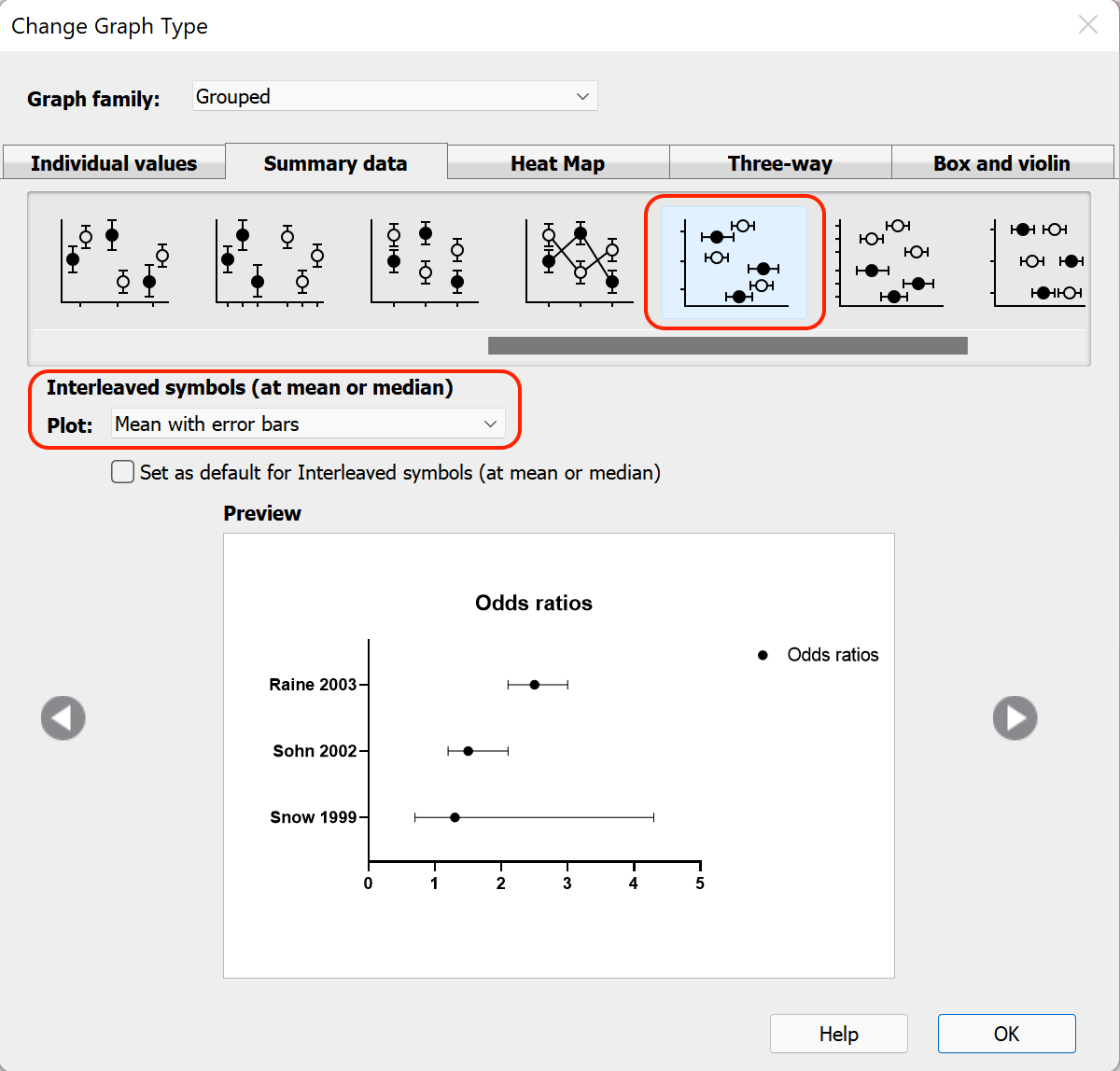The default graph should look something like this:Note that some meta-analysis plots use larger symbols to denote studies with more subjects. Prism can't currently apply symbol size settings to be proportional to a specified variable like this (except for multiple variable graphs). However, you can adjust the size of each symbol in the Format Graph dialog to make some larger and some smaller. Other steps that we'll take to customize this graph will be:

• Delete the Y axis title (the study names entered into the row titles of the data table should be displayed so that we don't need an axis title)
• Change the X axis title to "Odds ratio"
• Delete the Graph title and the graph legend

The next thing we may want to do is to add a custom vertical line at X=1 to indicate an odds ratio of 1 (when discussing odds ratios, an odds ratio of 1 represents "even odds" or "no effect"). To add such a line, double click on the X axis to bring up the Format Axes dialog (alternatively, you can click the "Format Axes" button in the Change section of the toolbar). The controls at the bottom of the "X axis" tab in the section "Additional ticks and grid lines" will allow us to add the desired line. We'll want to add our vertical line at a value of 1 on the X axis, so we enter this value in the "At X=" text box, and make sure that the "Line" box is checked.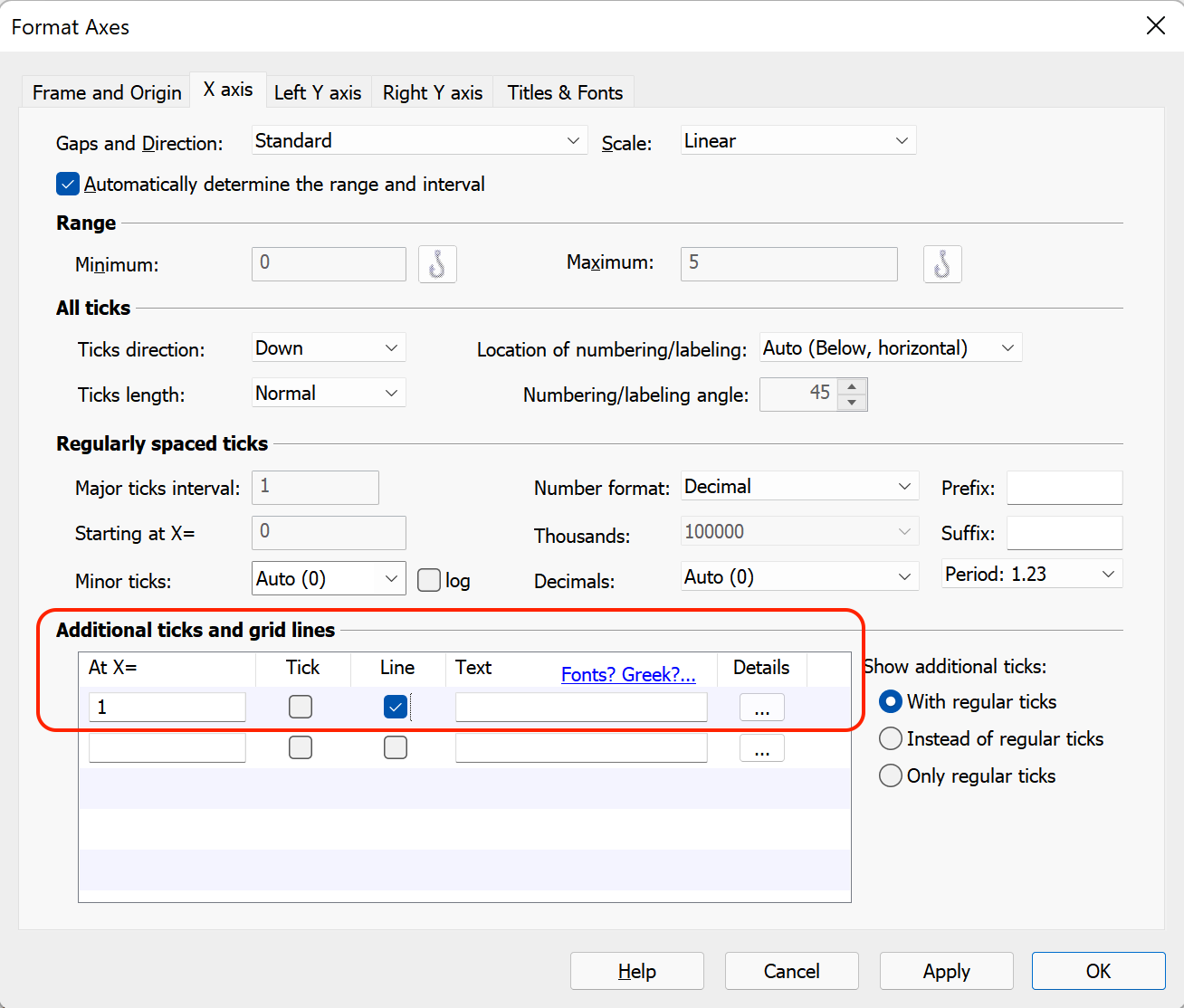When we click "OK", the graph should now have a vertical line at X=1, and should look like this:Often in fields such as epidemiology, these graphs will be plotted on a logarithmic axis instead of a linear axis. The result of this change is that odds ratios greater than 1.0 and odds ratios less than 1.0 become symmetrical. For example, on a logarithmic axis, an odds ratio of 2.0 and an odds ratio of 0.5 are the same distance away from an odds ratio of 1.0 (in other words, an odds of 2:1 and an odds of 1:2 are the same "distance" from an odds of 1:1, which should make sense).

Changing the scale of the X axis from linear to logarithmic in Prism is quite easy. To do this, simply open the Format Axes dialog again by double clicking on the X axis (or using the toolbar button). At the top of the "X axis" tab, there is a dropdown menu to change the axis "Scale". Use this dropdown menu to select Log 10 (or Log 2 or Ln depending on your preference). To make it very clear that a logarithmic axis is being used, we'll make a couple of changes in the "Regularly spaced ticks" section of this tab. Ensure that the "Number format" dropdown menu is set to "Antilog", and use the "Minor ticks" dropdown menu to select 9. Finally, ensure that the box for "log" is checked, and click "OK".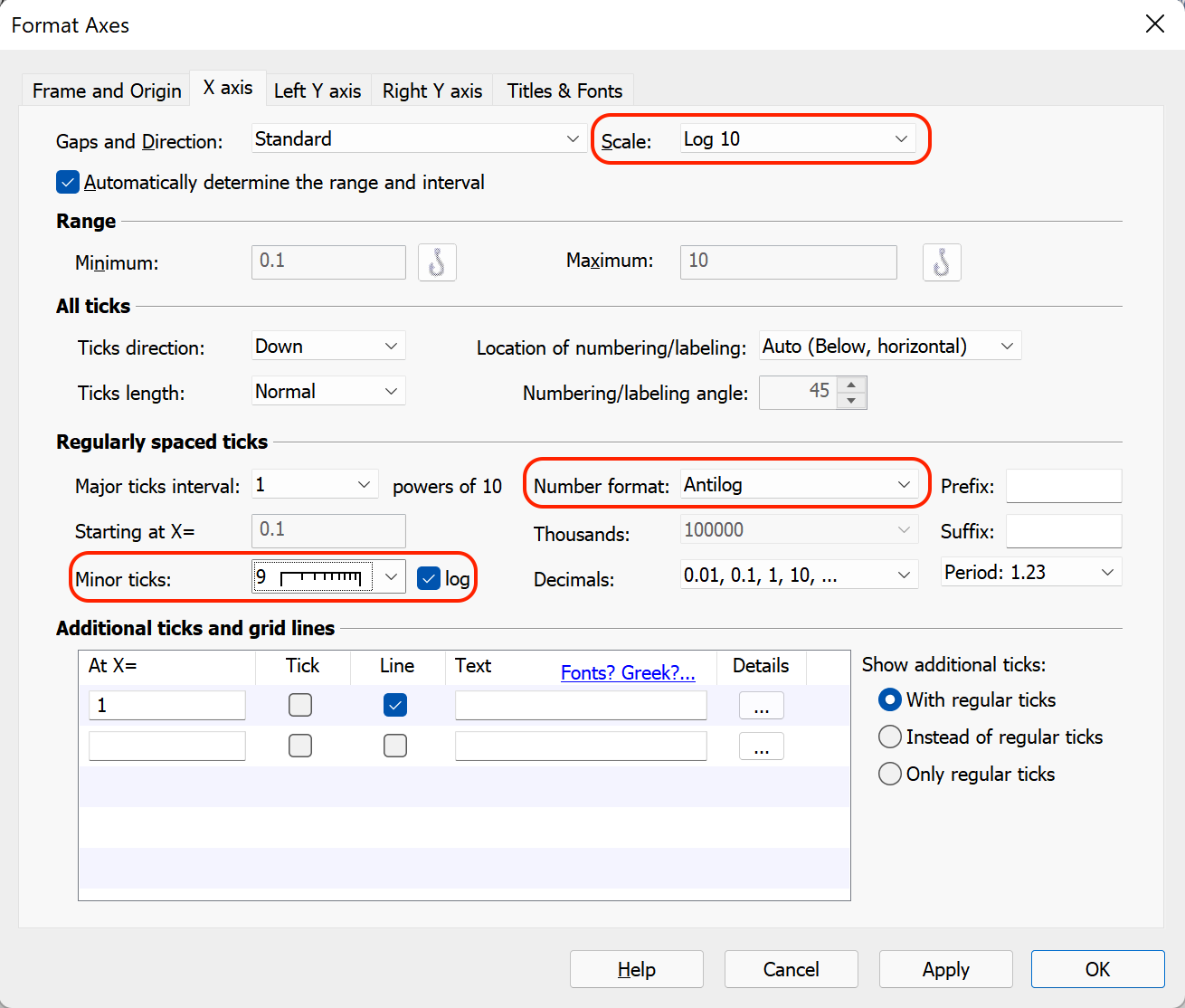Now the graph should look something like this:And you're done!